# Tutorial: diffusion in a simple liquid¶

## Prerequisites¶

You need a proper installation of HAL’s MD package and, for the analysis part, Python with the h5py package. The HDF5 tools (e.g., h5ls) are not required, but of advantage.

These simulation scripts are found in the folder /share/doc/halmd/examples relative to the installation path:

• liquid/lennard_jones_equilibration.lua
• liquid/lennard_jones.lua
• liquid/rescale_velocity.lua
• plotting/plot_h5md.py

## Equilibration phase¶

Melting of the initial lattice configuration with an NVT simulation:

halmd lennard_jones_equilibration.lua -v \
--output lj_thermalisation
--density 0.7 \
--temperature 1.3 \
--cutoff 2.5 \
--time 1e2 \
--sampling state-vars=200


Inspect the output and determine the mean potential energy from the second half:

h5ls -d lj_thermalisation.h5/observables/potential_energy
plot_h5md.py lj_thermalisation.h5
plot_h5md.py --no-plot --range 50 -1 lj_thermalisation.h5


You should obtain a potential energy per particle of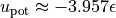, from which we calculate the internal (or: total) energy as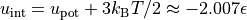.

Continue the simulation in the NVE ensemble, i.e., truly Newtonian dynamics, for a similar period of time. Before, we slightly rescale the velocities (< 1%) to match the average internal energy. And some expensive observables are turned off:

halmd lennard_jones.lua -v \
--output lj_equilibration \
--input lj_thermalisation.h5 \
--rescale-to-energy -2.007 \
--cutoff 2.5 \
--time 1e2 \
--sampling structure=0 correlation=0


Note

It is crucial to specify the cutoff here again. A better way would be to define the potential in a small lua file, which is included by both simulation scripts.

Let us check some thermodynamic properties again:

plot_h5md.py --no-plot --range 50 -1 lj_equilibration.h5


## Production phase¶

The same script is then used for production, with all sampling turned on:

halmd lennard_jones.lua -v \
--output lj_production \
--input lj_equilibration.h5 \
--cutoff 2.5 \
--time 1e3


No energy rescaling is applied here.

## Diffusion analysis¶

The diffusion constant can be obtained from the mean-square displacement (MSD) data,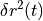. The latter are found in the output file lj_production.h5 of the last run at the following location within the data structure: dynamics/all/mean_square_displacement. The Python script provided at examples/plotting/diffusion_constant.py performs the analysis described in the following. An exemplary invocation that limits the fit range is:

diffusion_constant.py --range 0 -6 --min-time 100 lj_production.h5


The result for the diffusion constant should be in the range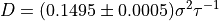.

For convenience, a command line interface is defined at the end of the analysis script. The result of the option parser is then passed to the main function.

        plt.show()

def parse_args():
import argparse

# define and parse command line arguments
parser = argparse.ArgumentParser()
parser.add_argument('--group', default='all', help='particle group in H5MD file')
parser.add_argument('--no-plot', action='store_true', help='do not produce plots, but do the analysis')
parser.add_argument('--rectify', action='store_true', help='rectify plot by showing MSD(t) / t')
parser.add_argument('--range', type=int, nargs=2, help='range of data points to include in fit')
parser.add_argument('--min-time', type=float, nargs=1, help='left end of time interval used for fitting')
parser.add_argument('input', metavar='INPUT', help='H5MD input file with MSD data')
return parser.parse_args()



The input file is passed as positional argument (without keyword). The main function begins by loading some libraries …

def main(args):
import h5py
import numpy as np
from scipy.optimize import curve_fit


… and reading the MSD data from the H5MD input file. The data are originally arranged in overlapping blocks of time grids of growing resolution. For our purpose, we flatten the arrays and bring them in chronological order first.

    with h5py.File(args.input, 'r') as H5:
msd = H5['dynamics/{0}/mean_square_displacement'.format(args.group)]

# get relavant data for MSD calculation
x = np.array(msd['time']).flatten()
y = np.array(msd['value']).flatten()
yerr = np.array(msd['error']).flatten()

# bring data in chronological order
idx = np.argsort(x)
x, y, yerr = x[idx], y[idx], yerr[idx]


The diffusion law,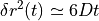, holds asymptotically only, and the data at long times are noisy. So we restrict the fit by evaluating the command-line options --range and --min-time and masking the data accordingly. The former specifies an index range [start, stop), and the latter gives the minimal lag time of the correlation.

    mask = np.ones_like(x, dtype=bool)

# deselect according to --range argument
if args.range:

# deselect according to --min-time argument, exclude t = 0 data
min_time = args.min_time or 0

# split input data


Eventually, we fit the selected data with a linear function that passes through the origin, and print the obtained diffusion constant along with an error estimate.

    dimension = args.dimension
linear_model = lambda x, D : 2 * dimension * D * x

popt, pcov = curve_fit(linear_model, fit_x, fit_y, sigma=fit_yerr)
perr = np.sqrt(np.diag(pcov))



If not deselected, we generate a log-log plot of the MSD data along with the obtained diffusion law. The data used in the fit is blue, whereas the other data is green. As an option, a more sensitive representation shows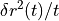, rectifying the expected linear increase.

    # generate plot
if not args.no_plot:
import matplotlib.pyplot as plt

# time grid for reference lines
t = np.logspace(np.log10(fit_x) - 1, np.log10(fit_x[-1]) + .3)

# plot MSD versus time
if not args.rectify:
plt.errorbar(fit_x, fit_y, yerr=fit_yerr, fmt='xb', label="data used in fit")
plt.errorbar(no_fit_x, no_fit_y, yerr=no_fit_yerr, fmt='xg', label="data not used in fit")
plt.loglog(t, linear_model(t, *popt), '-k')
ylabel = "Mean-square displacement"
else:
fit_rect = 2 * dimension * fit_x
no_fit_rect = 2 * dimension * no_fit_x
plt.errorbar(fit_x, fit_y / fit_rect, yerr=fit_yerr / fit_rect, fmt='xb', label="data used in fit")
plt.errorbar(no_fit_x, no_fit_y / no_fit_rect, yerr=no_fit_yerr / no_fit_rect, fmt='xg', label="data not used in fit")
plt.semilogx(t, linear_model(t, *popt) / (2 * dimension * t), '-k')
ylabel = "MSD(t) / {0:d}t".format(2 * dimension)

# add axes labels and finalise plot
plt.axis('tight')
plt.xlabel('Time')